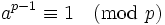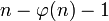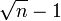# Fermat primality test

## Definition

The Fermat primality test is a quick and often inconclusive primality test. In the version below, we consider only.

Given a natural number, the test works as follows:

1. Pick a random elementfrom the congruence classes of nonzero integers mod(exclude the congruence class of 0). Explicitly, pickas an integer among.
2. Check whether. To compute the power use repeated squaring. If the condition does not hold, thenis composite. If the condition does hold, thenmay be prime or composite.

The above is a single iteration of the test. Multiple iterations of the test may be carried out with multiple random elements. If at any stage the conditionis violated, we can conclude that the number is composite. If, however, the condition holds every time, the situation is inconclusive andmay be prime or composite.

## Theory

### Why it works

The primality test above is correct because of Fermat's little theorem, which says that for a primeandrelatively prime to, we have:### Behavior on composite numbers

For any composite number, we use the following terms:

• A Fermat witness foris a valuesuch that.
• A Fermat liar foris a valuesuch that.
• We say thatis a Fermat pseudoprime to baseifis a Fermat liar for.

Nonzero numbers that have a common factor withalways serve as Fermat witnesses. There areof these whereis the Euler totient function. Forcomposite, this number is at least equal to, the worst case arising whenis a square of a prime. In general, ifhas a small number of large prime factors, there are very few such numbers and a uniform random choice is highly unlikely to hit them.

For the numbers that are relatively prime to, we have the formula for probability of relatively prime integer being a Fermat liar. If the probability is 1, thenis a Carmichael number. If the probability is less than 1, then it is at most 1/2, which means that the Fermat primality test can find a witness with arbitrarily high probability of success in a finite number of trials.